• 提示： \begin{align} 与 \begin{equation}不能同时使用 \begin{aligned} 可以与 \begin{equation}同时使用 ...多行公式编号 % 多行公式编号 \begin{align} \hat{C}_t &= tanh(W_C\...
目录
多行公式多编号
多行公式单编号，编号居中
多行公式无编号
多行公式多编号，有的行没有编号
一行公式分多行写
情况（case）划分
大括号单编号
大括号多编号

提示：
\begin{align} 与 \begin{equation}不能同时使用\begin{aligned} 只能与 \begin{equation}同时使用要想使用\begin{align}，需要在 \begin{document} 之前插入\usepackage{amsmath}\begin{align}的用法应该与\begin{gather}用法相同，区别是：
\begin{align}是默认右对齐，或者用 & 指定对齐位置\begin{gather} 默认是居中对齐，不能与 & 结合使用
概括：
导言区要加上，amsmath宏包如果想有编号，可以用gather，align如果想某一行没有编号，在用\\换行前使用\nonumber 如果不想有编号，可以gather*，align*，或者在后面加上命令\notag如果想一行公式分开写，用split如果想选择判断，用casesalign，split，cases 的公式对齐，用&

这里主要介绍使用 \begin{align}的公式编号，以LSTM的公式为例子：
多行公式多编号
% 多行公式多编号
\begin{align}
\hat{C}_t &= tanh(W_C\odot[h_{t-1},x_t]+b_C) \\
i_t&=\sigma(W_i\odot[h_{t-1},x_t]+b_i) \\
f_t&=\sigma(W_f\odot[h_{t-1},x_t]+b_f) \\
C_t&=f_t * C_{t-1}+i_t * \hat{C}_t \\
o_t&=\sigma(W_o\odot[h_{t-1},x_t]+b_o) \\
h_t&=o_t * tanh(C_t)
\end{align}
实现效果：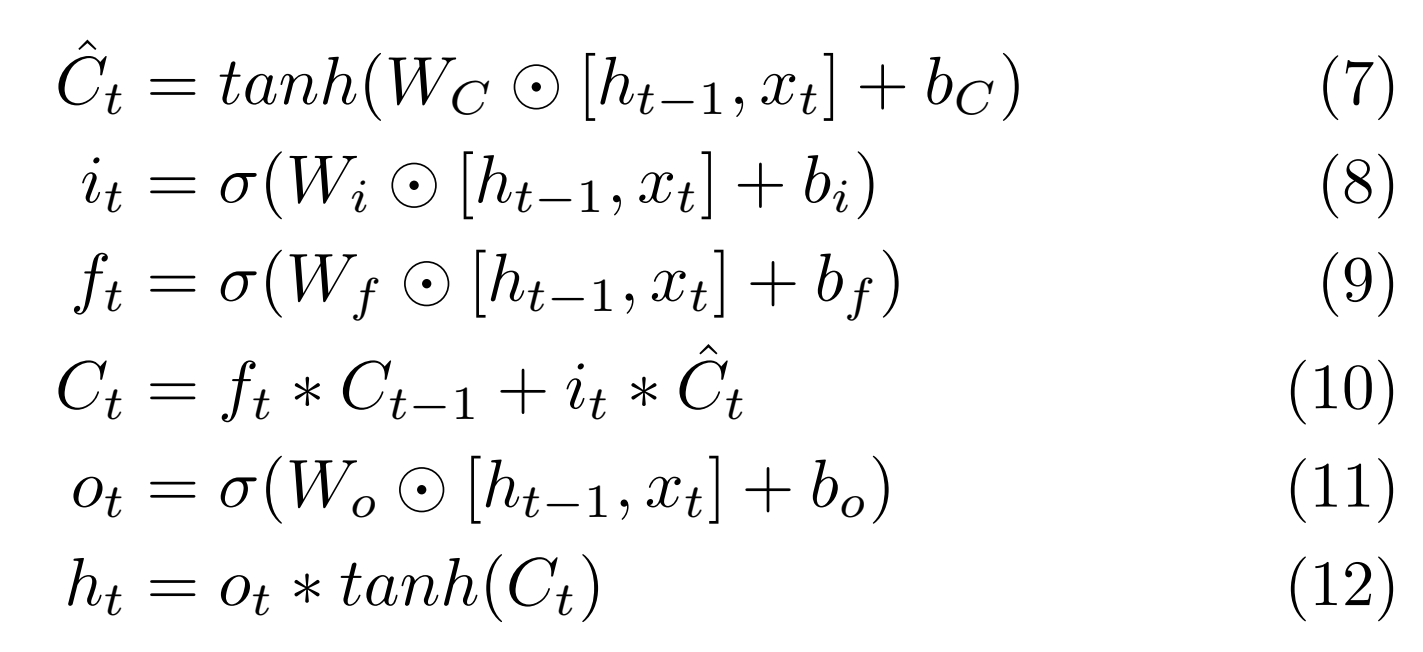多行公式单编号，编号居中
% 多行公式单编号
\begin{equation}
\begin{aligned}
&\hat{C}_t = tanh(W_C\odot[h_{t-1},x_t]+b_C) \\
&i_t=\sigma(W_i\odot[h_{t-1},x_t]+b_i) \\
&f_t=\sigma(W_f\odot[h_{t-1},x_t]+b_f) \\
&C_t=f_t * C_{t-1}+i_t * \hat{C}_t \\
&o_t=\sigma(W_o\odot[h_{t-1},x_t]+b_o) \\
&h_t=o_t * tanh(C_t)
\end{aligned}
\end{equation}
实现效果：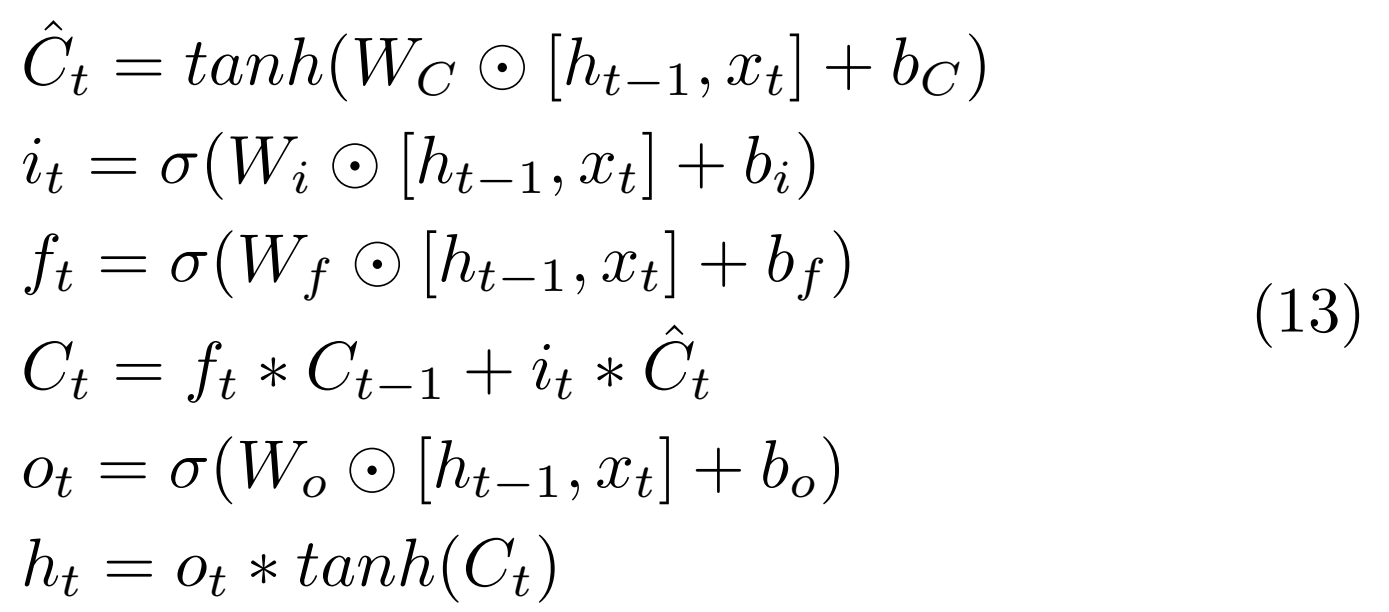多行公式无编号
% 多行公式无编号
\begin{align*}
\hat{C}_t &= tanh(W_C\odot[h_{t-1},x_t]+b_C) \\
i_t&=\sigma(W_i\odot[h_{t-1},x_t]+b_i) \\
f_t&=\sigma(W_f\odot[h_{t-1},x_t]+b_f) \\
C_t&=f_t * C_{t-1}+i_t * \hat{C}_t \\
o_t&=\sigma(W_o\odot[h_{t-1},x_t]+b_o) \\
h_t&=o_t * tanh(C_t)
\end{align*}
实现效果：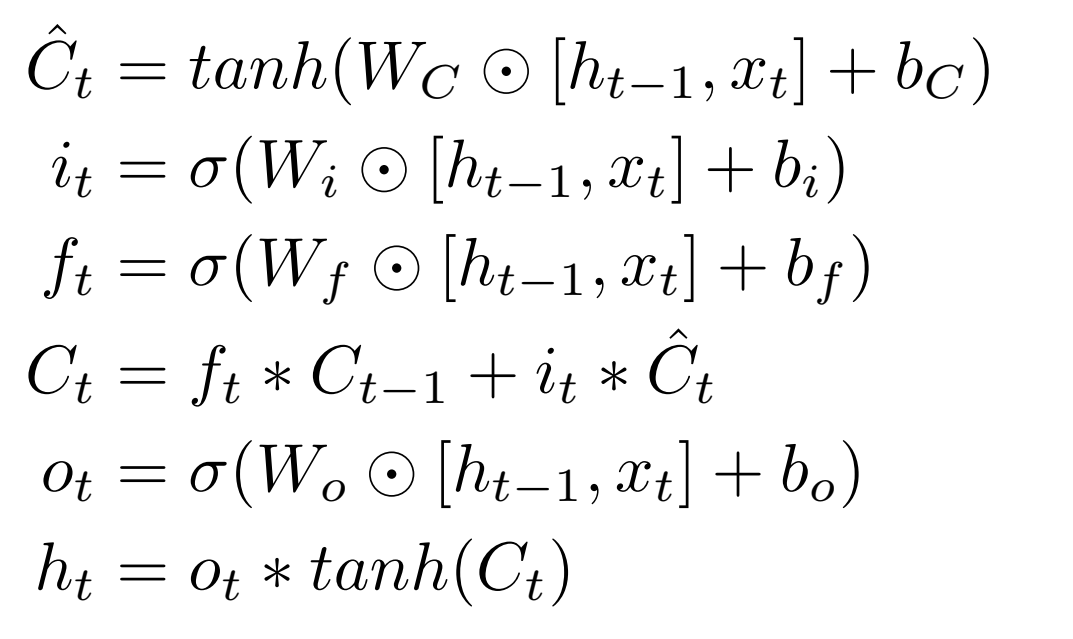多行公式多编号，有的行没有编号
% 多行公式多编号，有的行没有编号
\begin{align}
\hat{C}_t &= tanh(W_C\odot[h_{t-1},x_t]+b_C) \\
i_t&=\sigma(W_i\odot[h_{t-1},x_t]+b_i)  \nonumber \\
f_t&=\sigma(W_f\odot[h_{t-1},x_t]+b_f) \\
C_t&=f_t * C_{t-1}+i_t * \hat{C}_t \\
o_t&=\sigma(W_o\odot[h_{t-1},x_t]+b_o) \\
h_t&=o_t * tanh(C_t)
\end{align}
实现效果：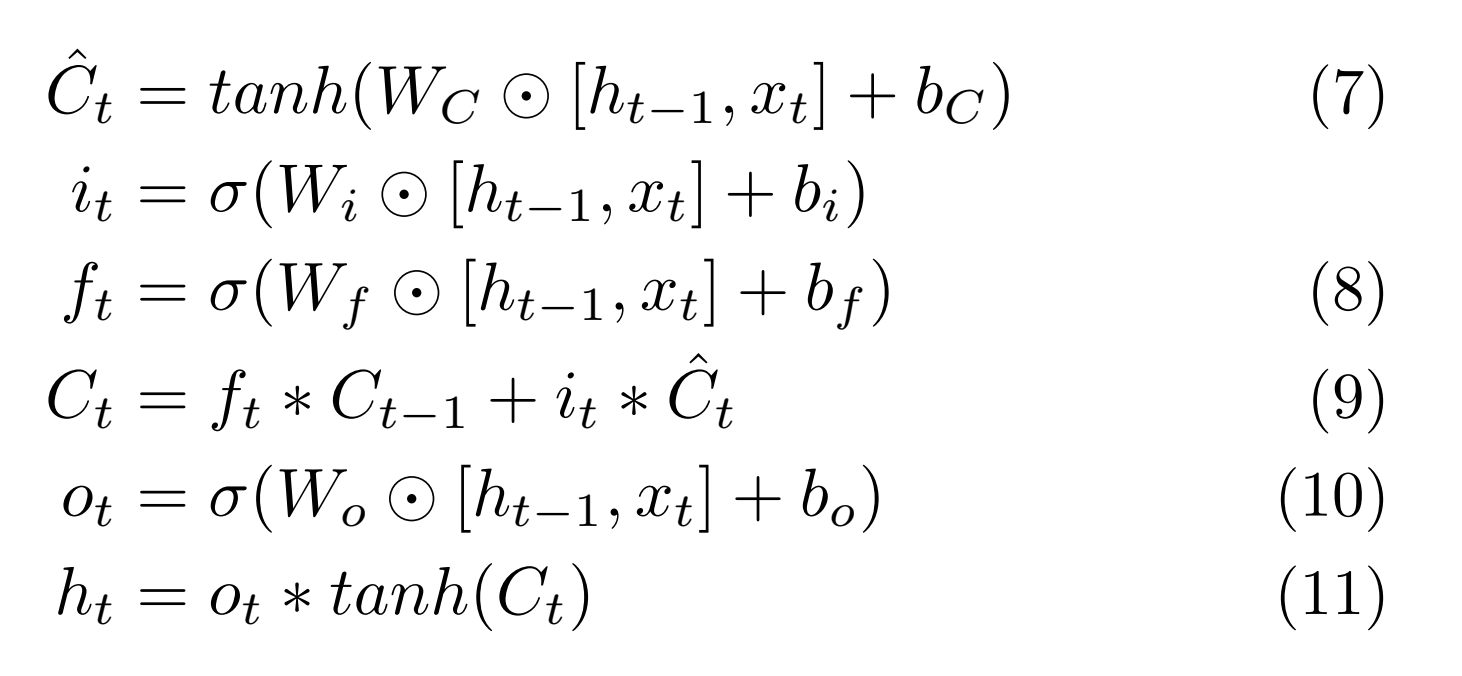一行公式分多行写
%split环境（用对齐）(一个公式分为多行排版) \begin{equation} \begin{split} \cos 2x &= \cos^2 x - \sin^2 x\\ &= 2\cos^2 x - 1 \end{split} \end{equation} 实现效果：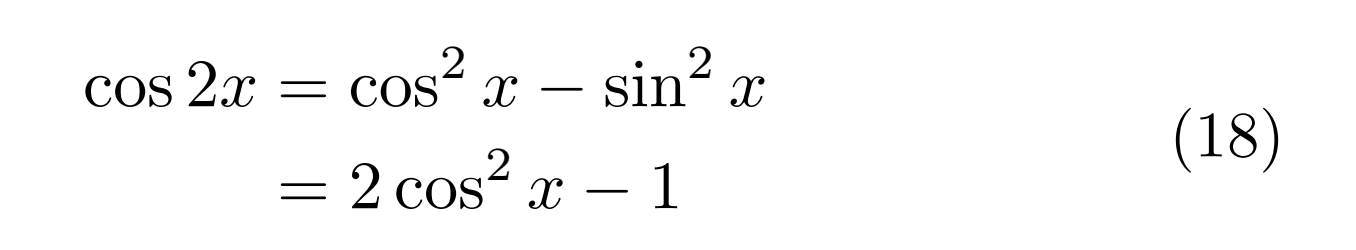情况（case）划分 \begin{equation} D(x) = \begin{cases} 1, & if \ x \in Q \\ 0, & if \ x \in R \end{cases} \end{equation} 实现效果：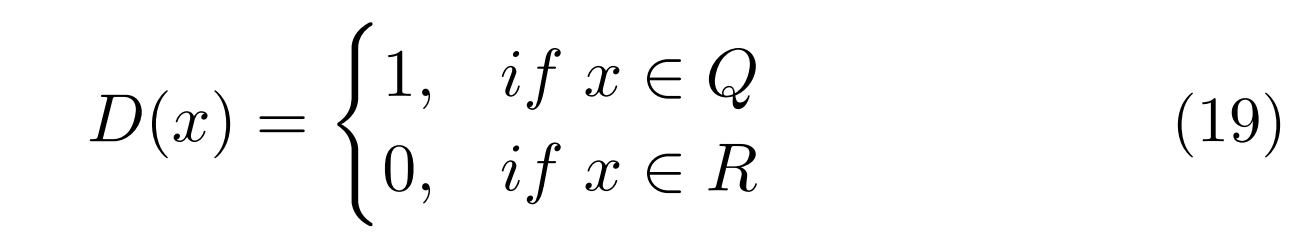大括号单编号 \begin{align} \left\{ %在equation环境下使用，用\left\{命令添加左大括号，用\right.以打点.结束 \begin{aligned} x&=eq1\\ y&=eq2+1 \end{aligned} \right. \end{align} 实现效果：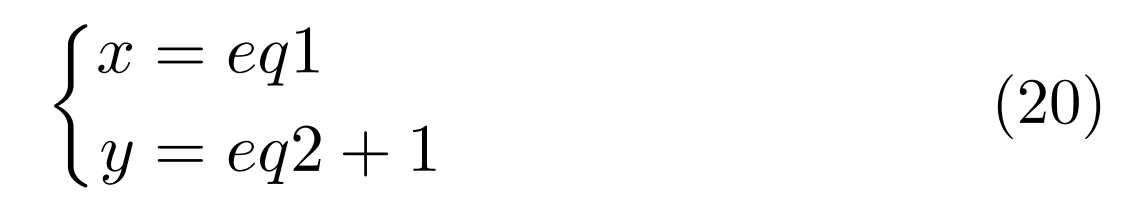大括号多编号 \usepackage{cases} % 大括号多编号 \begin{numcases}{} x_1&=eq1 \label{eqsystem1} \\ x_2+1&=eq2 \label{eqsystem2} \end{numcases} 实现效果：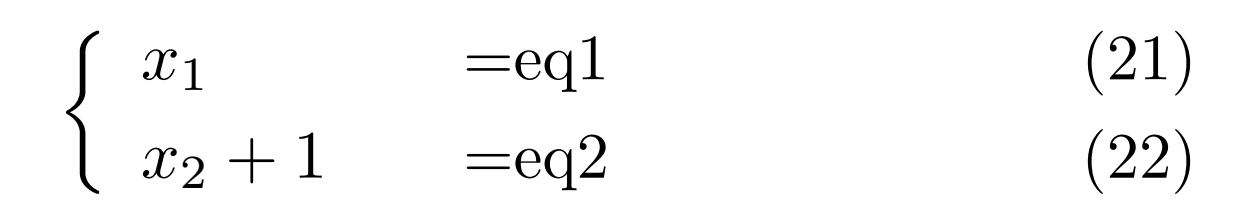更多大括号与编号的情况参考：latex多行公式加大括号、整体编号及多行编号及不同方法的区别 参考：latex12-LaTeX数学公式的多行公式（一）  展开全文• 1、编号多行的中间 代码：&表示公式对齐，\\是换行 2、每一行都加编号，但是可以选择哪一行不加 代码：包\usepackage{amsmath}，\nonumber加在前面表示该行不加编号 ... 1、编号在多行的中间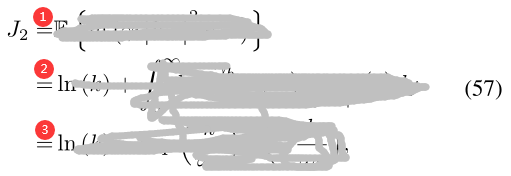代码：&表示公式对齐，\\是换行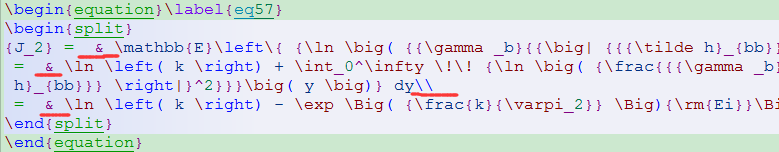2、每一行都加编号，但是可以选择哪一行不加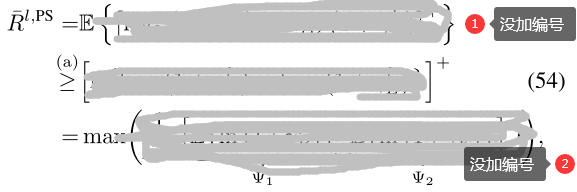代码：包\usepackage{amsmath}，\nonumber加在前面表示该行不加编号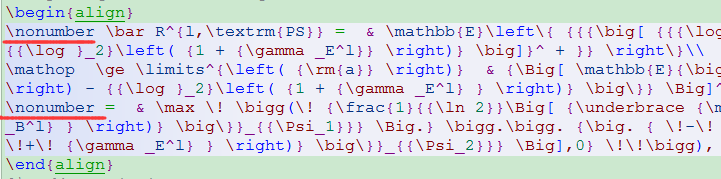展开全文• 目录单独加大括号并自动加整体编号，但不能为每行编号不能加大括号的多行编号带大括号，不会自动编号也不能加编号既要大括号也要多行编号多行编号后缀 单独加大括号并自动加整体编号，但不能为每行编号 \document... 环境：texlive2021，xelatex 目录 单独加大括号并自动加整体编号，但不能为每行编号不能加大括号的多行编号带大括号，不会自动编号也不能加编号既要大括号也要多行编号多行编号后缀不带大括号多行编号后缀 单独加大括号并自动加整体编号，但不能为每行编号 \documentclass[UTF8]{ctexart} \usepackage{amsmath} \begin{document} \begin{align}\left\{\begin{aligned} x&=eq1\\ y&=eq2+1 \end{aligned}\right.\end{align} \end{document}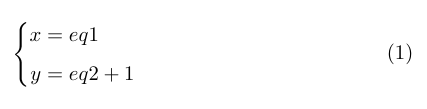不能加大括号的多行编号 \documentclass[UTF8]{ctexart} \usepackage{amsmath} \begin{document} \begin{align} x&=eq1 \label{eqsystem1} \\ y&=eq2+1 \label{eqsystem2} \end{align} \end{document}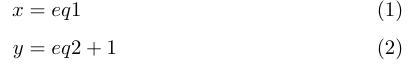其中\label用于其它地方的引用，可以省略。 带大括号，不会自动编号也不能加编号 \documentclass[UTF8]{ctexart} \usepackage{amsmath} \begin{document} $\begin{cases} x&=eq1 \\ y+1&=eq2 \end{cases}$ \end{document}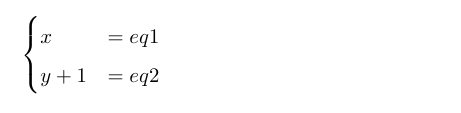既要大括号也要多行编号 \documentclass[UTF8]{ctexart} \usepackage{amsmath} \usepackage{cases} \begin{document} \begin{numcases}{} x_1&=eq1 \label{eqsystem1} \\ x_2+1&=eq2 \label{eqsystem2} \end{numcases} \end{document}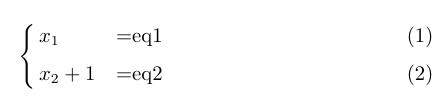多行编号后缀不带大括号 \documentclass[UTF8]{ctexart} \usepackage{amsmath} \begin{document} \begin{subequations}\label{AC0} \begin{align} x+y=z,\\ x+y+222+444+1=z,\\ 1+2=3. \end{align} \end{subequations} \end{document}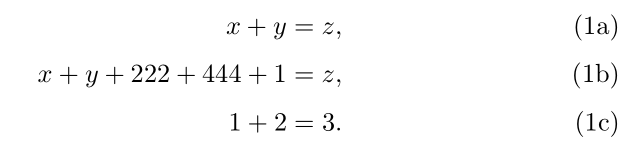从这里开始要使用caes宏包，可在命令行中使用texdoc cases命令查看。 多行编号后缀 \documentclass[UTF8]{ctexart} \usepackage{amsmath} \usepackage{cases} \begin{document} \begin{subnumcases} {\label{weqn} w\equiv} 0 & forc = d = 0$\label{wzero}\\ \sqrt{|c|}\,\sqrt{\frac{1 + \sqrt{1+(d/c)^2}}{2}} & for$|c| \geq |d|$\\ \sqrt{|d|}\,\sqrt{\frac{|c/d| + \sqrt{1+(c/d)^2}}{2}} & for$|c| < |d|$\end{subnumcases} Then, using$w$from eq.~(\ref{weqn}), the square root is \begin{subnumcases}{\label{sqrteqn} \sqrt{c+id}=} 0\,, &$w=0$(case \ref{wzero})\\ w+i\frac{d}{2w}\,, &$w \neq 0$,$c \geq 0$\\ \frac{|d|}{2w} + iw\,, &$w \neq 0$,$c < 0$,$d \geq 0$\\ \frac{|d|}{2w} - iw\,, &$w \neq 0$,$c < 0$,$d < 0$\end{subnumcases} \end{document}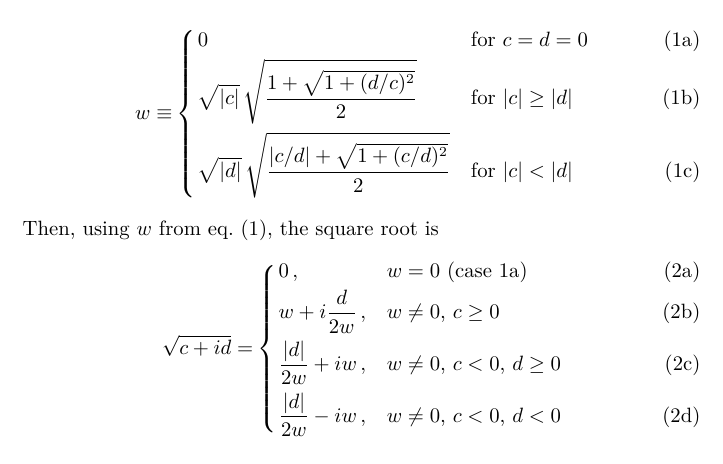展开全文• 基础 首先介绍内联公式和单行公式，内联公式使用单$，单行公式使用双。 我定义了一个函数： y=axy =axy=...多行公式 推荐使用aligned环境，他还有个兄弟align，基本用法如下 \begin{aligned} f(x) &= ax + b \ 基础 首先介绍内联公式和单行公式，内联公式使用...，单行公式使用$...$。 我定义了一个函数： y = a x y =ax 我定义了一个函数： y = a x y = ax 这一种用法在latex里是没有自动编号的。 equation环境 \begin{equation} y=ax \end{equation}  这一种用法在latex是可以自动编号的。 多行公式 推荐使用aligned环境，他还有个兄弟align，基本用法如下 \begin{aligned} f(x) &= ax + b \\ &= b + ax \end{aligned}$$ $$\begin{align} f(x) &= ax + b \\ &= b + ax \end{align} 需要注意 aligned 需要在 math mode 下使用，也就是需要加  他们两者的区别就在编号上，aligned是没有自动编号的，而align是每行都有编号。这里我们会有这样两种需求 1、希望只有一个编号居中，那么请使用 \begin{equation} \begin{aligned} f(x) &= ax + b \\ &= b + ax \end{aligned} \end{equation}  2、希望编号在最后一行公式，那么请使用 \begin{align} f(x) &= ax + b \nonumber \\ &= b + ax \end{align}  其中 \nonumber 让那行的编号消失了 3、如果想让公式自动居中，那么就使用 gather 环境 \begin{gather} f1 = ax + b \\ f2 = b + ax \end{gather}  展开全文• Typora中使用LaTeX多行公式左对齐 有时候公式太长，用=号对齐很难看(有的公式左边很长，右边很短)，此时难免需要进行"公式左对齐"。所需要的环境还是"align"(或者是align*，不带公式编号)。 语法如下： \begin{... • 其右端的编号(1)如何显示在两个等式的中间？ 利用split环境可以将编号写在中间，如： begin{equation} begin{split} a+b & =1\ c+d & =2 end{split} end{equation} 加入label： ... • \begin{equation} \label{eqn2} \begin{split} n&=\left[\frac{b-a}{0.01}\right]+1, \\ S&=\frac{1}{n}\sum\limits_{j=1}^{n}(\lambda_{0j}-\lambda_{j}). \end{split} \end{equation} • 公式组环境中，使用取消序号命令来编排多行公式，将序号置于最后一行末尾，代码如下： \begin{align} f(x) =& 2(x + 1)^{2}-1\nonumber\\ =& 2x^{2} + 4x + 1 \end{align} 结果如下： 如果想要将... • ## Latex公式及编号 千次阅读 2017-04-07 09:31:14 Latex公式编号 1、latex公式编号 如果用eqnarray环境，只能将编号显示在上面或下面等式的后面，如： \begin{eqnarray} a+b = 1 \\ c+d = 2 \end{eqnarray} 编号显示在第二个等式的后面。 ... • Latex多行公式左对齐 (2011-06-27 13:09:59) 转载▼ 标签： latex 多行公式 左对齐 it 分类： 学习资料 今天想要用latex输入多行公式，参照LATEX一书... • 3.11 Latex使用笔记 ...多行公式 不需要序号的时候，在使用gather*命令，前后都要改 \begin{gather} \end{gather} 带左弯号的多行公式 \begin{equation} \left\{ %在equation环境下使用 \{ 命令... • 如果没有的话，这篇文章的公式类型很多：LaTeX技巧207：使用align环境输入多行公式的技巧 导言区要加上，amsmath宏包 如果想有编号，可以用gather，align 如果不想有编号，可以gather*，align*，或者在后面... • 1. 罗列多行公式 2. 拆分单个公式 3. 将公式组合成块 1. 罗列多行公式 基础语法： 1.gather 环境 ：可以在\\ 前使用\notag 命令阻止指定行不编号； \begin{gather} a + b = b + a \\ ... • 最近在用Latex写东西，遇到了多行对齐公式的输入与编号问题，尝试了网上的许多方法，最终的解决方法记录如下： 方法一：前边\usepackage{amsmath}，利用equation，内部嵌套split，则会在公式的最后一行进行编号； ...抽象代数 • LaTeX数学公式多行公式 %导言区 \documentclass{ctexart} %ctexbook ctexrep %\usepackage{ctex} \usepackage{amsmath} \usepackage{amssymb} %正文区 \begin{document} %可以使用gather环境实现多行公式... • %导言区 \documentclass{ctexart} %ctexbook ctexrep %\usepackage{ctex} \usepackage{amsmath} ... %可以使用gather环境实现多行公式排版 %内部可以使用\\换行 %带编号 \begin{gather} a + b =... • 今天想要用latex输入多行公式，参照LATEX一书中的\begin{eqnarray}equation\\equation\\...\\equation\end{eqnarray}发现无法左对齐，对我当中的省略号造成了很不好的影响，因此在网上搜了下多行公式左对齐的方法，... • ## Latex公式编号问题 万次阅读 多人点赞 2019-12-25 21:37:43 目录对某个公式编号/不编号 在写文章时，我们会遇到各种各样的对公式编号的要求，...latex中给我们提供了很多编辑公式的方法，具体可在终端（命令提示符窗口）输入如下命令查看官方文档，这里我们做简单总结。 ... • 编号 [code="Latex"] \begin{align*} p(f_1=0) &= 0.8/0.9*p(s_1=0)+0.1/0.9*p(s_2=0) \\ &= 0.8/0.9*0.4+0.1/0.9*0.6 \\ &= 0.4222 \end{align*} [/code] [img]... • ## Latex公式与编号 千次阅读 2013-04-22 17:24:08 LaTeX的数学公式有两种，即行中公式和独立公式。行中公式放在正文中间，独立公式单独成行。 行中公式可以用如下这三种方法表示：\begin{math}x^n+y^n=z^n\end{math} ，或者 $$x^n+y^n=z^n$$ ，或者x^n+y^n
• LaTeX的数学公式有两种，即行中公式和独立公式。行中公式放在正文中间，独立公式单独成行。（1）行中公式可以用如下这三种方法表示： \begin{math}x^n+y^n=z^n\end{math}\begin{math}x^n+y^n=z^n\end{ma...
• 1.自动编号的单行公式环境是 \begin{equation} … \end{equation} 不参与自动编号的单行公式环境： $…$ 人工编号的单行公式可以使用Tex原有的行间公式标记 $$公式 \eqno 编号$$ 将编号放在右边 ...
• LaTeX数学公式的矩阵、多行公式 目录 矩阵公式 数学公式多行公式 矩阵公式 % 导言区 \documentclass{article} \usepackage{ctex} \usepackage{amsmath} %矩阵宏包 % 创建新命令，自定义省略号类型 \new...
• 本文的主要内容有：如何输入行内公式如何输入行间公式多行公式常用的数学符号输入方法相信观察力敏锐的同学一定已经注意到在上一次的推送里的这段代码：\documentclass[a4paper,12pt,landscape,twocolumn]{book}\...
• % 每行公式中使用 & 分隔为两部分 % 通常表示值和后面的条件 \begin { equation } D ( x ) = \begin { cases } 1, & \text { 如果 } x \in \mathbb { Q } ; \\ 0, & \text { 如果 } x \in \......